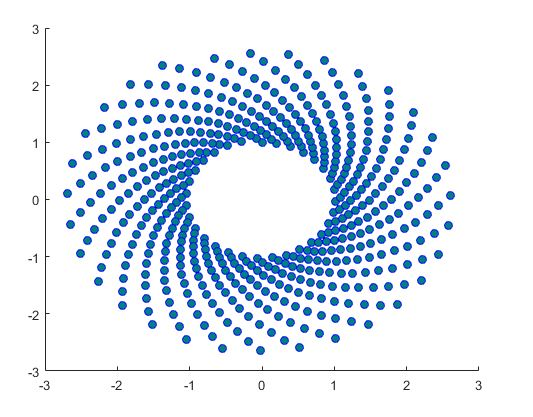# Matlab中散点图绘制详细教程scatter函数（附matlab代码）

## scatter函数绘制散点图

``````scatter(x,y)
scatter(x,y,sz)
scatter(x,y,sz,c)
scatter(___,'filled')
scatter(___,mkr)
scatter(___,Name,Value)
scatter(ax,___)
s = scatter(___)
``````

① scatter(x,y) 在向量 x 和 y 指定的位置创建一个包含圆形的散点图。该类型的图形也称为气泡图。
② scatter(x,y,sz) 指定圆大小。要绘制大小相等的圆圈，请将 sz 指定为标量。要绘制大小不等的圆，请将 sz 指定为长度等于 x 和 y 的长度的向量。
③ scatter(x,y,sz,c) 指定圆颜色。要以相同的颜色绘制所有圆圈，请将 c 指定为颜色名称或 RGB 三元组。要使用不同的颜色，请将 c 指定为向量或由 RGB 三元组组成的三列矩阵。
④ scatter(,‘filled’) 填充圆形。可以将 ‘filled’ 选项与前面语法中的任何输入参数组合一起使用。
⑤ scatter(
,mkr) 指定标记类型。
scatter(,Name,Value) 使用一个或多个名称-值对组参数修改散点图。例如，‘LineWidth’,2 将标记轮廓宽度设置为 2 磅。
⑥ scatter(ax,
) 将在 ax 指定的坐标区中，而不是在当前坐标区中绘制图形。选项 ax 可以位于前面的语法中的任何输入参数组合之前。
⑦ s = scatter(___) 返回 Scatter 对象。在创建散点图后，以后可使用 s 对其进行修改。

## 1、创建散点图

``````x = linspace(0,3*pi,200);
y = cos(x) + rand(1,200);
scatter(x,y)
``````## 2、改变圆圈大小

``````x = linspace(0,3*pi,200);
y = cos(x) + rand(1,200);
sz = linspace(1,100,200);
scatter(x,y,sz)
``````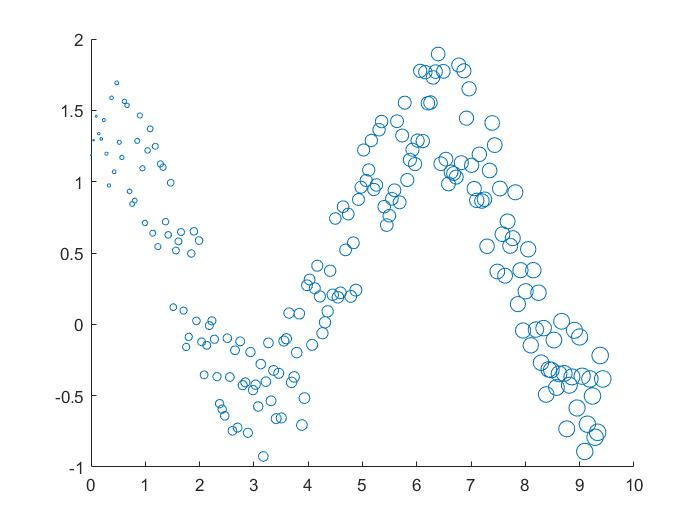x、y 和 sz 中的相应元素确定每个圆圈的位置和大小。要按照相同的面积绘制所有圆圈，请将 sz 指定为数值标量。

## 3、改变圆圈颜色

``````x = linspace(0,3*pi,200);
y = cos(x) + rand(1,200);
c = linspace(1,10,length(x));
scatter(x,y,[],c)
``````x、y 和 c 中的相应元素确定每个圆形的位置和颜色。scatter 函数将 c 中的元素映射到当前颜色图中的元素。

## 4、填充标记

``````x = linspace(0,3*pi,200);
y = cos(x) + rand(1,200);
sz = 25;
c = linspace(1,10,length(x));
scatter(x,y,sz,c,'filled')
``````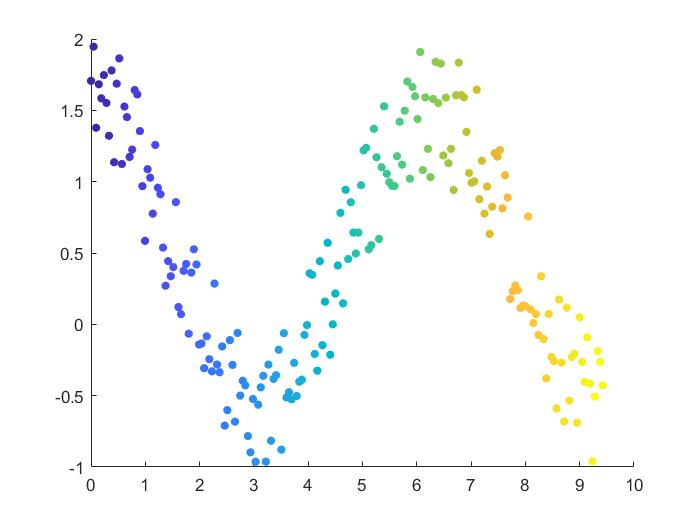## 5、指定标记符号

``````theta = linspace(0,2*pi,150);
x = sin(theta) + 0.75*rand(1,150);
y = cos(theta) + 0.75*rand(1,150);
sz = 140;
scatter(x,y,sz,'d')
``````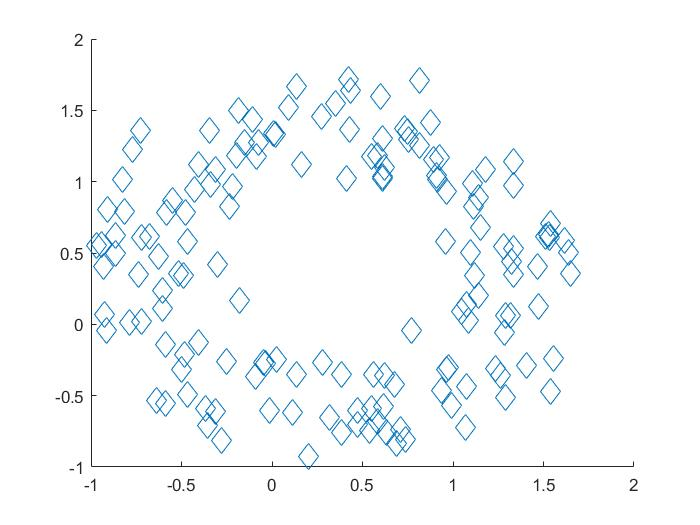## 6、更改标记颜色和线条宽度

``````theta = linspace(0,2*pi,300);
x = sin(theta) + 0.75*rand(1,300);
y = cos(theta) + 0.75*rand(1,300);
sz = 40;
scatter(x,y,sz,'MarkerEdgeColor',[0 .5 .5],...
'MarkerFaceColor',[0 .7 .7],...
'LineWidth',1.5)
``````## 7、指定散点图的子图

``````x = linspace(0,3*pi,200);
y = cos(x) + rand(1,200);
ax1 = subplot(2,1,1);
scatter(ax1,x,y)

ax2 = subplot(2,1,2);
scatter(ax2,x,y,'filled','d')
``````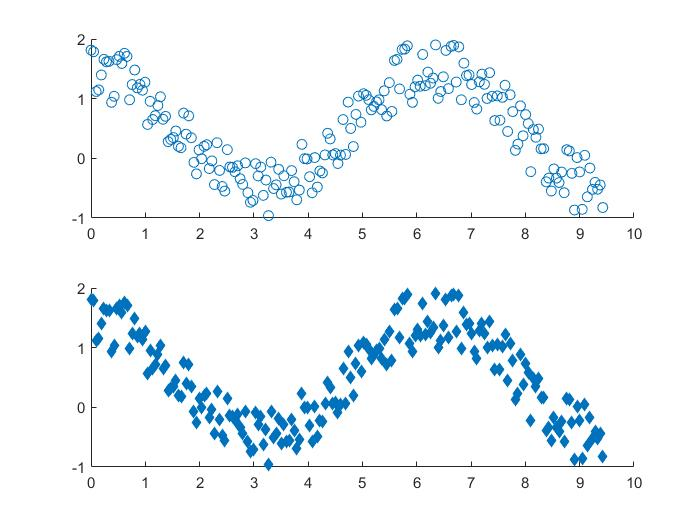## 8、创建并修改散点序列

``````theta = linspace(0,1,500);
x = exp(theta).*sin(100*theta);
y = exp(theta).*cos(100*theta);
s = scatter(x,y);
````````````s.LineWidth = 0.6;
s.MarkerEdgeColor = 'b';
s.MarkerFaceColor = [0 0.5 0.5];

``````